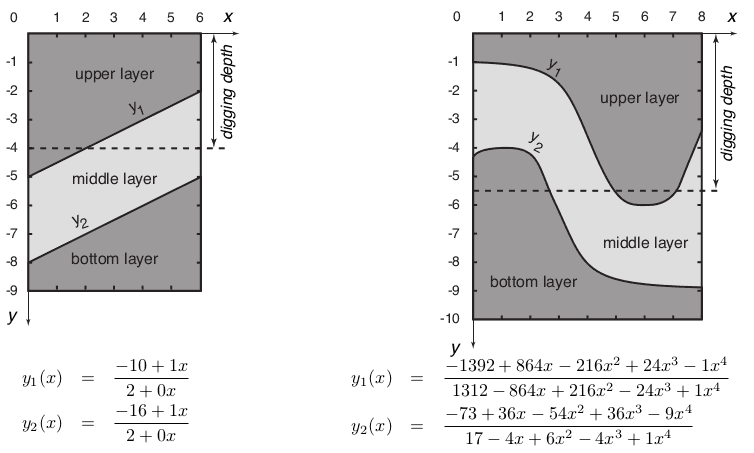### E - Environment Protection

##### Languages: C, C++, Java, Pascal, ... (details)

Arsenic & Cyanide Mining (ACM) is a corporation that has recently decided to start developing its mines in the lands near your hometown. As a member of the citizen’s regulatory committee for ACM’s operations, your task is to control how much the corporation can mine from those lands, so that you get to keep the jobs and other benefits without sacrificing the environment and the health of the local residents.

The ACM has plans to mine several rectangular patches of land. A patch of land has width $W$, can be dug up to a maximum depth $D$, and has a flat surface which we consider to be at depth $0$.The minerals in a patch are organized in three layers, which may vary in their depth along the width of the patch, but always have the same profile along its whole length. This is why the ACM is only interested in the profile along the width of each patch, and has performed exploratory work in order to precisely determine its shape. As a result, they discovered that the two interfaces between the three layers of minerals can be represented by two functions $y_1(x)$ and $y_2(x)$, where the first describes the boundary between the top layer and the middle layer, and the second describes the boundary between the middle layer and the bottom layer. These functions are always such that

$−D \lt y_2(x) \lt y_1(x) \lt 0$ for $0 \leq x \leq W$,

so that the layers’ boundaries never touch each other. Besides, each function has the form $y_i(x) = p_i(x) / q_i(x)$, where

$p_i(x) = \sum_{k=0}^{K} P_{i,k}x^k$ and $q_i(x) = \sum_{k=0}^{K} Q_{i,k}x^k$,

for $i = 1, 2$ and a certain integer $K$. The figure below shows the profiles of two patches of land in the way the ACM represents them. The patch on the left has width $W = 6$ and depth $D = 9$, while the patch on the right has $W = 8$ and $D = 10$. The boundaries of the layers of each patch are described by the functions defined below them.The ACM will dig everything in a patch of land up to a certain digging depth d, and then sell all the minerals thus obtained to make a profit. However, the minerals in the top and the bottom layers are essentially worthless, so the profit of the whole operation comes exclusively from those minerals in the middle layer. In fact, the profit is proportional to the area $A$ of the middle layer in the profile that is at depth at most $d$. Given the description of a patch of land and an integer $A$, you would like to know the digging depth $d$ you should allow the ACM to dig the patch so that they get an area of minerals from the middle layer in the profile of exactly $A$. In the figure above you can see the answer for the two test cases in the sample input. For the patch on the left, in order to get an area $A = 4$ the digging depth must be $d = 4.00000$, while for the patch on the right an area $A = 14$ requires a digging depth $d = 5.51389$.

#### Input

Each test case is described using five lines. The first line contains four integers $W$, $D$, $A$ and $K$, where $W$ is the width of the patch of land the ACM wants to mine $(1 \leq W \leq 8)$, $D$ is its depth $(1 \leq D \leq 10)$, $A$ is the area of the middle layer in the profile that the ACM must get $(1 \leq A \leq W \times D)$, and $K$ allows the definition of the interfaces $y_1(x)$ and $y_2(x)$ as explained above $(0 \leq K \leq 8)$. Each of the other lines contains $K + 1$ integers between $−10^8$ and $10^8$, inclusive. The second line contains the coefficients of $p_1(x)$ from $P_{1,0}$ to $P_{1,K}$. The third line contains the coefficients of $q_1(x)$ from $Q_{1,0}$ to $Q_{1,K}$. The fourth line contains the coefficients of $p_2(x)$ from $P_{2,0}$ to $P_{2,K}$. The fifth line contains the coefficients of $q_2(x)$ from $Q_{2,0}$ to $Q_{2,K}$ . Within each test case A is strictly less than the total area of the middle layer in the profile, and there exists a single value $d$ such that a digging depth $d$ yields an area of minerals from the middle layer in the profile of exactly $A$. Besides, $q_1(x) \ne 0$, $q_2(x) \ne 0$ and $−D \lt y_2(x) \lt y_1(x) \lt 0$, for $0 \leq x \leq W$.

#### Output

For each test case output a line with a rational number representing the depth d that the ACM should be allowed to dig the patch of land so that they get an area of minerals from the middle layer in the profile of exactly $A$. The result must be output as a rational number with exactly five digits after the decimal point, rounded if necessary.

#### Sample test(s)

Input
6 9 4 1 -10 1 2 0 -16 1 2 0 8 10 14 4 -1392 864 -216 24 -1 1312 -864 216 -24 1 -73 36 -54 36 -9 17 -4 6 -4 1
Output
4.00000 5.51389